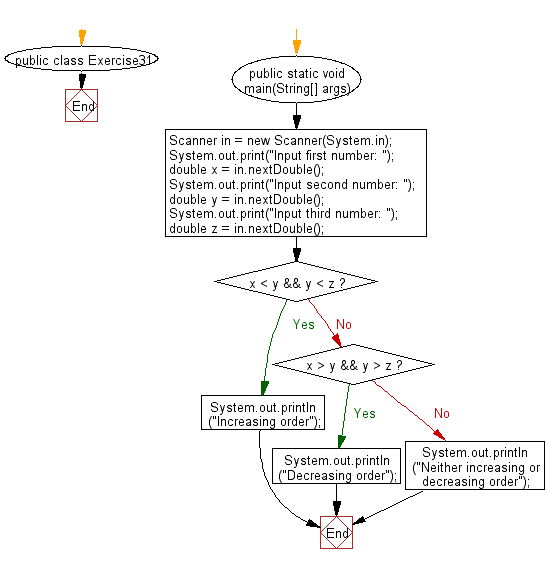﻿ Java exercises: Accepts three numbers from the user and prints - w3resource# Java Conditional Statement Exercises: Accepts three numbers from the user and prints

## Java Conditional Statement: Exercise-31 with Solution

Write a program that accepts three numbers from the user and prints "increasing" if the numbers are in increasing order, "decreasing" if the numbers are in decreasing order, and "Neither increasing or decreasing order" otherwise.

Test Data
Input first number: 1524
Input second number: 2345
Input third number: 3321

Sample Solution:

Java Code:

``````import java.util.Scanner;
public class Exercise31 {
public static void main(String[] args)
{
Scanner in = new Scanner(System.in);
System.out.print("Input first number: ");
double x = in.nextDouble();
System.out.print("Input second number: ");
double y = in.nextDouble();
System.out.print("Input third number: ");
double z = in.nextDouble();
if (x < y && y < z)
{
System.out.println("Increasing order");
}
else if (x > y && y > z)
{
System.out.println("Decreasing order");
}
else
{
System.out.println("Neither increasing or decreasing order");
}
}
}
```
```

Sample Output:

```Input first number: 1524
Input second number: 2345
Input third number: 3321
Increasing order
```

Flowchart:Java Code Editor: